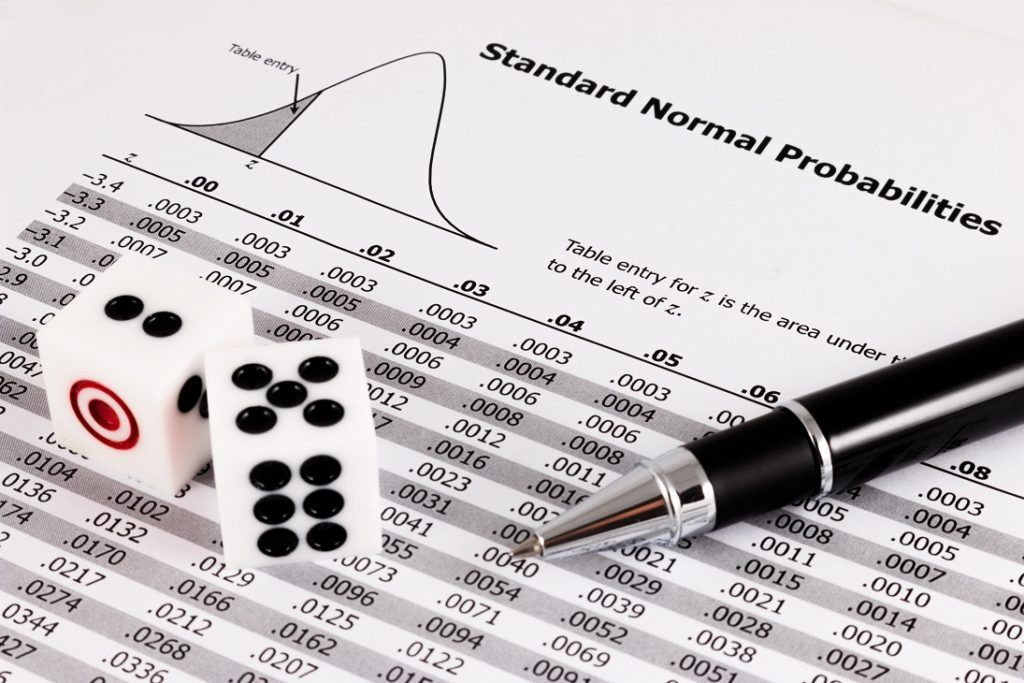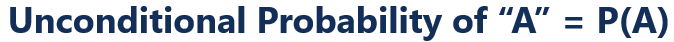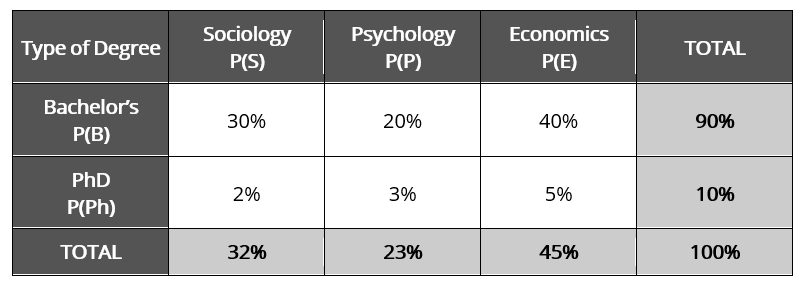# Unconditional Probability

A probability that is unaffected by previous or future events

## What is Unconditional Probability?

Unconditional probability, also known as marginal probability, refers to a probability that is unaffected by previous or future events. In other words, unconditional probability is the probability of an event regardless of the preceding or future occurrence of other events. In simplest terms, unconditional probability is simply the probability of an event occurring.### Summary:

• Unconditional probability refers to a probability that is unaffected by previous or future events.
• The unconditional probability of event “A” is denoted as P(A).
• A conditional probability, contrasted to an unconditional probability, is the probability of an event of which would affect or be affected by another event.

### Formula for Unconditional ProbabilityTherefore, the unconditional probability of an event happening is simply the probability of the event itself. An unconditional probability does not have a condition.

For example, the probability of rain tomorrow in itself is an unconditional probability.

Further examples of unconditional probabilities:

• What is the probability of a dice rolling a 6?
• What is the probability of landing a head on a coin toss?
• What is the probability of drawing an ace of spades in a deck of cards?

### Unconditional Probability vs. Conditional Probability

A conditional probability, contrasted to an unconditional probability, is the probability of an event of which would affect or be affected by another event. In other words, a conditional probability, as the name implies, comes with a condition.

For example, recall the following unconditional probability: “What is the probability of rain tomorrow?” A conditional probability can be phrased as follows: “What is the probability of rain tomorrow, given today is sunny?”

Further examples of conditional probabilities:

• What is the probability of rolling a 6 followed by a 4?
• What is the probability that it is sunny today given that it rains tomorrow?
• What is the probability of the S&P 500 hitting all-time highs and then consolidating at 3,000?

### Example of Unconditional Probability

The following joint probability table shows the distribution of Social Science students in a Bachelor’s and Ph.D. programs:Using your understanding of unconditional probability, determine:

1. The unconditional probability of randomly selecting a student who is pursuing a bachelor’s degree;
2. The unconditional probability of randomly selecting a student who is studying Sociology;
3. The unconditional probability of randomly selecting a student who is pursuing a Ph.D.; and
4. The unconditional probability of randomly selecting a student who is studying Economics.

1. P(B) = 90%
2. P(S) = 10%
3. P(Ph) = 10%
4. P(E) = 45%

### Unconditional Probability in the News

Unconditional probability can be commonly found in the news. For example, according to a CNBC article, the CME Group’s FedWatch tool showed traders pricing in a 100% probability of an interest rate cut in July 2019. It, in itself, is an example of an unconditional probability!